Maths - Notation

Multidimensional Algebra

notation meaning basis Alternativedirect sum or Kronecker sum depending on contextdirect product or Kronecker product depending on context
/\ exterior algebrascalar or real depending on context (see below) e0² 2 dimensional vector e1,e2³ 3 dimensional vector e1,e2,e3n n dimensional vector e1…en² bivector based on 2D algebra e12³ bivector based on 3D algebra e12,e31,e23
C complex numbersH quaternions³
O octonions7
aA a is an element of the set A

Scalar

I have used the term 'scalar' interchangeably with the term 'real', that is, a continuous value that can be represented by a single number.

Strictly speaking the term 'scalar' should be reserved for a quantity that is used to scale a vector, that is change its magnitude without changing its direction, or in other words a scalar is the ratio of parallel vectors.

For instance I should not really call energy a scalar because there are no vectors involved.

I apologise for my lack of mathematical rigor here, its just that the word scalar seems to better express that it is not a vector and its less likely to cause confusion with the real part of a complex number. Also this (mis?)usage is quite common in the computer world.

Matrix

notation meaning
[M] matrix
[M]t transpose of matrix (swap rows & columns)
[M]-1 inverse of matrix
[I] identity matrix (ones on leading diagonal, otherwise zeros)

The individual elements of the matrix are numbered as follows,

 m00 m01 m02 … m0n m10 m11 m12 … m1n m20 m21 m22 … m2nmp0 mp1 mp2 … mpn

The first subscript represents the row, the second subscript represents the column

Tensor Notation

notation example meaning pages in site
subscript e1 coordinate basis
superscript x1 coordinate value
Einstein Summation Convention eixi=e1x1+e2x2+e3x3=∑eixi When the same index appears twice in an expression, once raised and once lowered, a sum is implied.
partial derivatives a=∂/∂a

Set Theory

notation example meaning
A A = {a | aA} set
a aA element of A
{a, b, c}   set containing a, b and c
a H = {ah | hH} left coset of a
(a,b)   cycle notation
<a,b>   all the elements of group generated by a and b
<r,f | r³=1, f²=1, frf=r-1> generators with constrains
 K
K = {k | kK} complement of set indicated by bar over top
| A |   grade (size) = number of elements in A
| G : H |   number of left cosets of H in G
×   Direct ProductSemidirect Product (left){n, h}φ {n',h'} Semidirect Product (right)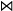Bicrossed Product
also known as Knit or Zappa-Szep product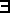h = h'H such that
a h' = h a
Normal subgroup a H = H a for all a in G
Ø   empty set
¬   not
and (= intersection)
or (U = union)is in (is an element of the set)AB A is contained in B (or A is a subset of B)
if
<->   if and only if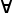for allthere existsis isomorphic to(S)   The set of subsets of S (power set)
orb(s)   Orbit - set of elements that can be reached
stab(s)   Stabilizer - set of elements that don't move s

Definition

Endo is a Greek word meaning inside.

Logic / Proofs

notation example meaning pages in site
/\

conjunction, and , intersection (in set), greatest lower bound (in order)

\/   disjunction, or , union (in set), least upper bound (in order)
T   Top (true)
_|_   Bottom (false)
=> A => B Implication
A => B means A implies BTφ

φ is a theorem of T
that is: φ is deducible from T

In contrast to 'implication' above this is a statement about statements usually written only when we mean φ follows from T, wheras A => B is a statement we may consider as a conjecture . See [Lawvere,Rosebrugh page 198]

Not to be confused with right adjoint in category theory.

logicfor all (quantifier)there exists (quantifier)

Category Theory

notation example meaning pages in site
AB   Map / Morphism / Function between mathematical structures that relates or preserves the structures in some way. May be injection, surjection or bijection (injection can be specifically indicated see below)
ab   A function in terms of elements of the structures aA, bBA function that is valid if all the functions drawn as solid lines are valid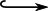injective morphism (embedding)canonical map - map of G onto factor group G/H where H is a normal subgroup of G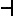Left adjointRight adjoint

Denotional Semantics

notation example meaning pages in site
[[D]] [[2*5]] = 10 A 'denotion' is written in double square brackets.
It represents the meaning of D to a program
evaluation of DBottom (undefined)Less defined than

Greek Alphabet

upper lower   upper lower   upper lower
Alpha A α Iota I ι Rho P ρ
Beta B β Kappa K κ Sigma Σ σ
Gamma Γ γ Lambda Λ λ Tau Τ τ
Delta Δ δ Mu M μ Upsilon Υ υ
Epsilon E ε Nu N ν Phi Φ φ
Zeta Z ζ Xi Ξ ξ Chi X χ
Eta H η Omicron O ο Psi Ψ ψ
Theta Θ θ Pi Π π Omega Ω ω

Various Operator Symbols

 × ÷ ± • ° ð Ð ƒ µ Ø ø Þ þ † … ∂ ◊ ≤ ≥ ∈ ≠ ≈ ∫ ∞ ∀ ∃ √

Powers of 10

 m 10-3 K 103 µ 10-6 M 106 n 10-9 G 109 p 10-12 T 1012 f 10-15 a 10-18